Last updated: 2019-01-11

• R Markdown file: uncommitted changes The R Markdown file has unstaged changes. To know which version of the R Markdown file created these results, you’ll want to first commit it to the Git repo. If you’re still working on the analysis, you can ignore this warning. When you’re finished, you can run wflow_publish to commit the R Markdown file and build the HTML.

• Environment: empty

Great job! The global environment was empty. Objects defined in the global environment can affect the analysis in your R Markdown file in unknown ways. For reproduciblity it’s best to always run the code in an empty environment.

• Seed: set.seed(12345)

The command set.seed(12345) was run prior to running the code in the R Markdown file. Setting a seed ensures that any results that rely on randomness, e.g. subsampling or permutations, are reproducible.

• Session information: recorded

Great job! Recording the operating system, R version, and package versions is critical for reproducibility.

• Repository version: dd9d56a

Great! You are using Git for version control. Tracking code development and connecting the code version to the results is critical for reproducibility. The version displayed above was the version of the Git repository at the time these results were generated.

Note that you need to be careful to ensure that all relevant files for the analysis have been committed to Git prior to generating the results (you can use wflow_publish or wflow_git_commit). workflowr only checks the R Markdown file, but you know if there are other scripts or data files that it depends on. Below is the status of the Git repository when the results were generated:

Ignored files:
Ignored:    .Rhistory
Ignored:    .Rproj.user/
Ignored:    analysis/.DS_Store
Ignored:    analysis/.httr-oauth
Ignored:    code/.DS_Store
Ignored:    code/differential_expression/
Ignored:    code/differential_phase/
Ignored:    data/
Ignored:    docs/.DS_Store
Ignored:    docs/figure/.DS_Store
Ignored:    docs/figure/neighboring_genes.Rmd/.DS_Store
Ignored:    output/compare/
Ignored:    output/ctss_clustering/
Ignored:    output/differential_detection/
Ignored:    output/differential_expression/
Ignored:    output/differential_phase/
Ignored:    output/extensive_transcription/
Ignored:    output/final_utrs/
Ignored:    output/gcbias/
Ignored:    output/homopolymer_analysis/
Ignored:    output/neighboring_genes/
Ignored:    output/promoter_architecture/
Ignored:    output/tfbs_analysis/
Ignored:    output/transcript_abundance/

Untracked files:
Untracked:  _workflowr.yml
Untracked:  docs/figure/tfbs_analysis.Rmd/
Untracked:  figures/

Unstaged changes:
Modified:   analysis/_site.yml
Modified:   analysis/analyze_neighboring_genes.Rmd
Modified:   analysis/array_correlations.Rmd
Modified:   analysis/calculate_transcript_abundance.Rmd
Deleted:    analysis/chunks.R
Modified:   analysis/comparing_utrs.Rmd
Modified:   analysis/ctss_clustering.Rmd
Modified:   analysis/dynamic_tss.Rmd
Modified:   analysis/extensive_transcription.Rmd
Modified:   analysis/final_utrs.Rmd
Modified:   analysis/gcbias.Rmd
Modified:   analysis/index.Rmd
Modified:   analysis/process_neighboring_genes.Rmd
Modified:   analysis/promoter_architecture.Rmd
Modified:   analysis/strain_differential_detection.Rmd
Modified:   analysis/strain_differential_expression.Rmd
Modified:   analysis/strain_differential_phase.Rmd
Modified:   analysis/tfbs_analysis.Rmd
Modified:   code/differential_detection/detect_transcripts.R
Modified:   code/figures.R
Deleted:    docs/Rplots.pdf


Note that any generated files, e.g. HTML, png, CSS, etc., are not included in this status report because it is ok for generated content to have uncommitted changes.
Expand here to see past versions:
File Version Author Date Message
Rmd fafe120 Philipp Ross 2018-10-01 finished dynamic tsss
Rmd f0b9974 Philipp Ross 2018-09-23 update to neighboring gene analysis
html f0b9974 Philipp Ross 2018-09-23 update to neighboring gene analysis
Rmd f59e2e3 Philipp Ross 2018-09-22 hellooooo
html f59e2e3 Philipp Ross 2018-09-22 hellooooo

## Overview

What is the relationship between the distances between neighboring genes and their co-expression? Can we identify putative bidirectional promoters this way? Are convergent neighboring genes that overlap more likely to be expressed at different time points?

## Analysis

First let’s read in the data we generated during processing the data:

# abundance estimates
# Without UTR predictions
all_neighboring <- dplyr::bind_rows(convergent,divergent,tandem)
# 3D7 UTR predictions
# HB3 UTR predictions
# IT UTR predictions
xit_divergent  <- readr::read_tsv("../output/neighboring_genes/it_divergent.tsv",col_names=TRUE)

### Before and after UTR predictions

Let’s make some plots of the before and after picture of distance between genes and their correlation to one another. Before we do this we need to actually generate the data we care about. We need to calculate the neighboring genes and the distances beween those genes. Then we can import that data, calculate the correlations between those neighboring genes, and create a gene-by-gene table of neighboring genes, the distances between them, their orientations, and the correlations between their expression patterns.

Should we remove genes for which we don’t have UTR predictions?

### Correlation by distance plots

We also want to filter out genes for which we have no 5’ or 3’ UTR predictions

utrs_3d7 <- tibble::as_tibble(rtracklayer::import.gff3("../output/final_utrs/final_utrs_3d7.gff"))
utrs_3d7$Parent <- unlist(utrs_3d7$Parent)

utrs_hb3 <- tibble::as_tibble(rtracklayer::import.gff3("../output/final_utrs/final_utrs_hb3.gff"))
utrs_hb3$Parent <- unlist(utrs_hb3$Parent)

utrs_it <- tibble::as_tibble(rtracklayer::import.gff3("../output/final_utrs/final_utrs_it.gff"))
utrs_it$Parent <- unlist(utrs_it$Parent)

And genes for which we actually detect a confident level of transcription:

# filter out genes with a TPM below the threshold
# and that are not protein coding genes
pcg <- tibble::as_tibble(rtracklayer::import.gff3("../data/annotations/PF3D7_codinggenes_for_bedtools.gff"))$ID get_filtered_ids <- function(abund,tpm_threshold) { fabund <- abund %>% dplyr::group_by(gene_id) %>% dplyr::summarise(f=sum(TPM>=tpm_threshold)) %>% dplyr::ungroup() %>% dplyr::filter(f>0 & gene_id %in% pcg) return(fabund$gene_id)
}
fx3d7 <- get_filtered_ids(x3d7_abund,5)
fxhb3 <- get_filtered_ids(xhb3_abund,5)
fxit  <- get_filtered_ids(xit_abund,5)

First we should look at some randomly sampled neighboring genes to get an idea of what the average level of correlatino between genes is:

set.seed(33)
random_cor <- sapply(seq(1,1000), function(x) {all_neighboring %>%
dplyr::filter(left_gene %in% utrs_3d7[utrs_3d7$type == "5UTR",]$Parent &
left_gene %in% utrs_3d7[utrs_3d7$type == "3UTR",]$Parent &
right_gene %in% utrs_3d7[utrs_3d7$type == "5UTR",]$Parent &
right_gene %in% utrs_3d7[utrs_3d7$type == "3UTR",]$Parent &
left_gene %in% fx3d7 & right_gene %in% fx3d7) %>%
dplyr::sample_n(1000,replace=F) %$% mean(cor)}) random_neighboring <- tibble::tibble(left_gene=NA,right_gene=NA,dist=NA,cor=random_cor,orientation="random") filtered_divergent <- divergent %>% dplyr::filter(left_gene %in% utrs_3d7[utrs_3d7$type == "5UTR",]$Parent & right_gene %in% utrs_3d7[utrs_3d7$type == "5UTR",]$Parent & left_gene %in% fx3d7 & right_gene %in% fx3d7) %>% dplyr::mutate(orientation="divergent") filtered_convergent <- convergent %>% dplyr::filter(left_gene %in% utrs_3d7[utrs_3d7$type == "3UTR",]$Parent & right_gene %in% utrs_3d7[utrs_3d7$type == "3UTR",]$Parent & left_gene %in% fx3d7 & right_gene %in% fx3d7) %>% dplyr::mutate(orientation="convergent") filtered_neighboring <- dplyr::bind_rows(filtered_convergent,filtered_divergent,random_neighboring) %>% dplyr::mutate(orientation=factor(orientation,levels=c("divergent","convergent","random"))) We can visualize this either as a density plot or boxplot: g <- filtered_neighboring %>% ggplot(aes(x=cor,group=orientation,color=orientation)) + geom_line(stat="density",size=1.5) + scale_color_brewer(palette="Accent") + ylab("Density") + xlab(expression(rho)) + theme(legend.position="bottom") print(g)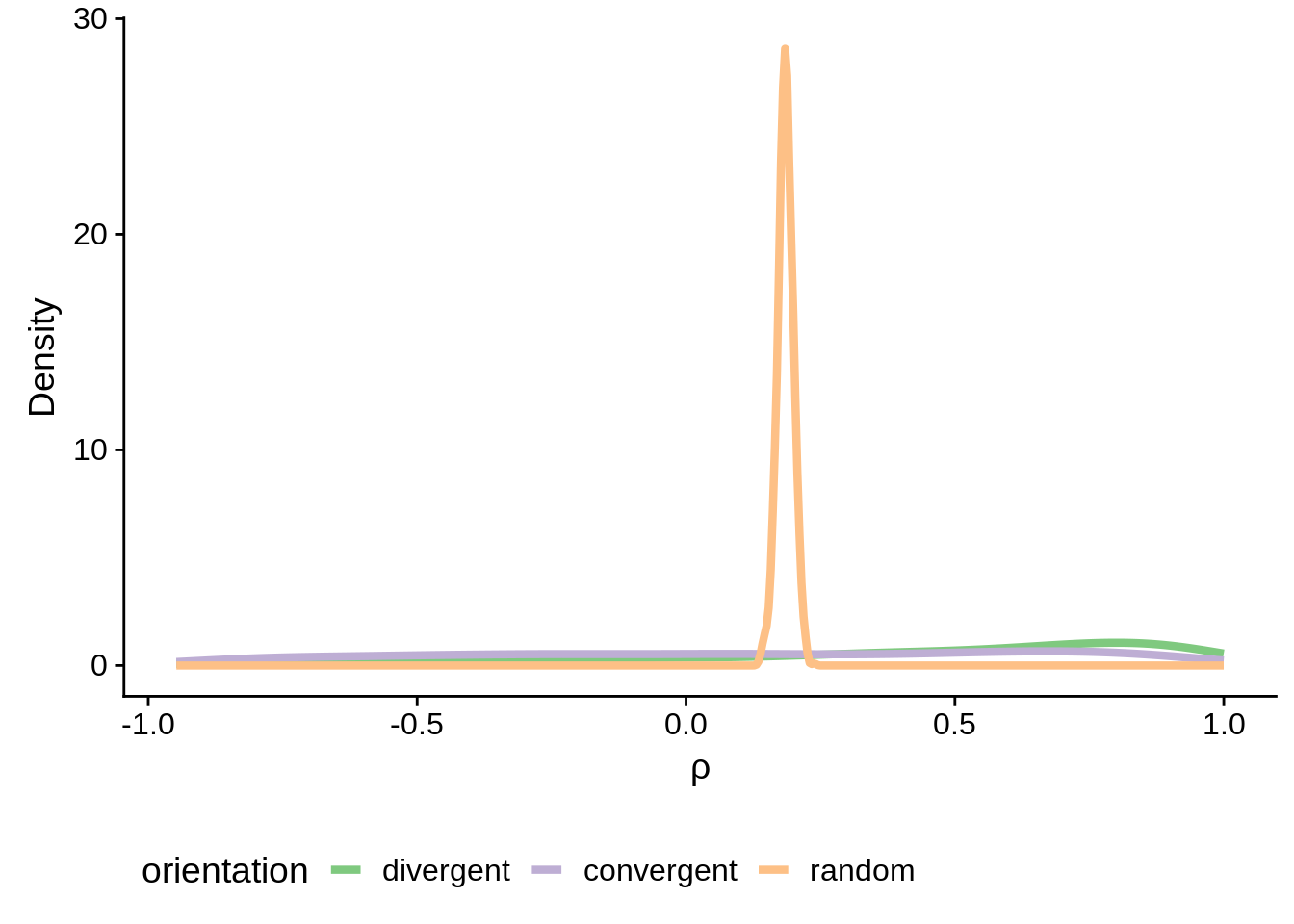ggsave(plot=g,filename="../output/neighboring_genes/neighboring_cor_density.svg",heigh=3,width=4) g <- filtered_neighboring %>% ggplot(aes(x=orientation,y=cor,fill=orientation)) + geom_boxplot(size=1) + scale_fill_brewer(palette="Accent") + ylab(expression("Correlation ("~rho~")")) + xlab("") + theme(axis.text.x = element_text(angle=60, hjust=1)) print(g)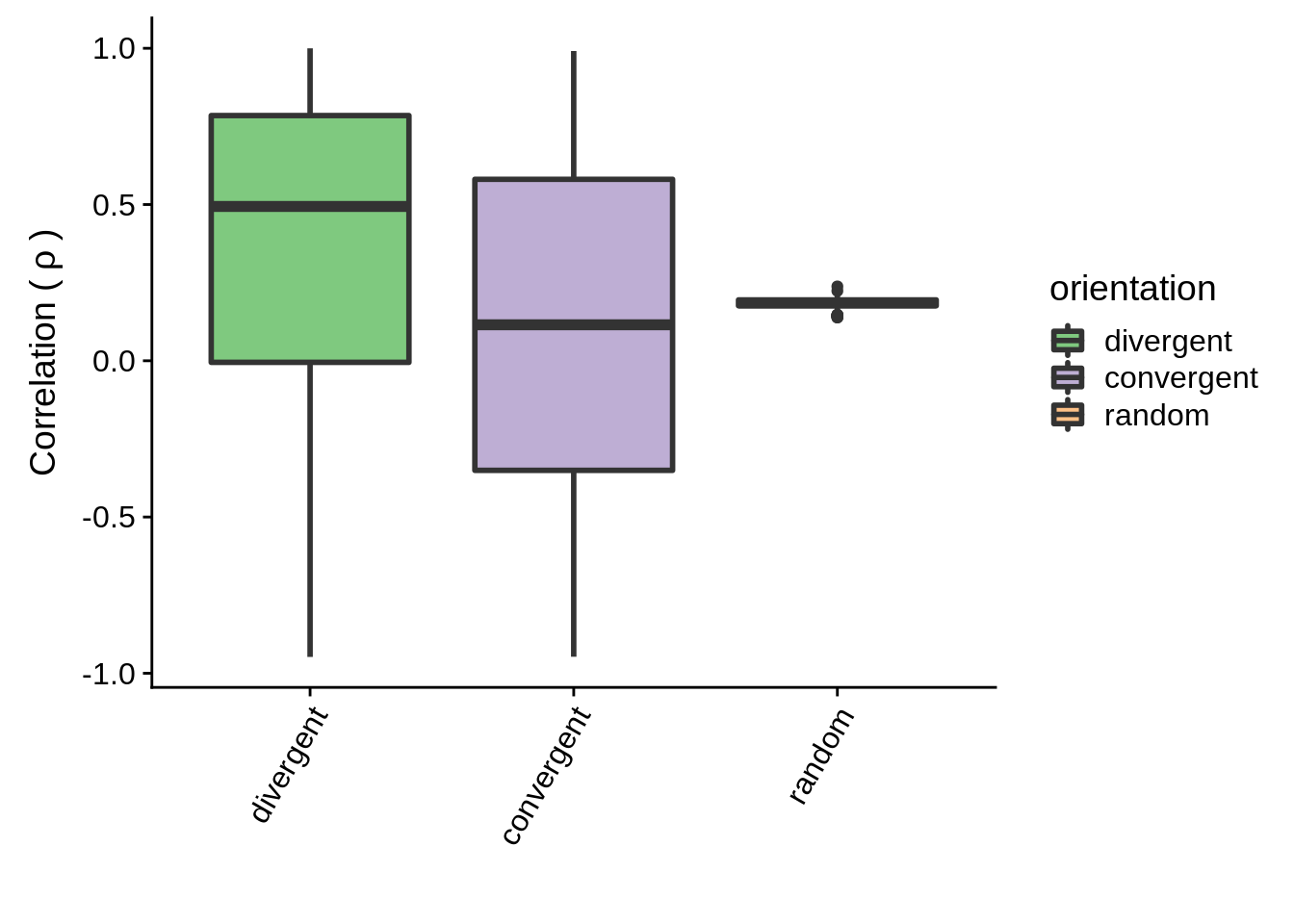ggsave(plot=g,filename="../output/neighboring_genes/neighboring_cor_boxplot.svg",heigh=3,width=4) Are the convergent and divergent correlations significantly different than random pairs? wilcox.test(filtered_divergent$cor,random_neighboring$cor)  Wilcoxon rank sum test with continuity correction data: filtered_divergent$cor and random_neighboring$cor W = 759400, p-value < 2.2e-16 alternative hypothesis: true location shift is not equal to 0 wilcox.test(filtered_convergent$cor,random_neighboring$cor)  Wilcoxon rank sum test with continuity correction data: filtered_convergent$cor and random_neighboring$cor W = 490430, p-value = 0.00376 alternative hypothesis: true location shift is not equal to 0 Now let’s filter the rest of the neighboring genes for the full-transcript distances: fx3d7_divergent <- x3d7_divergent %>% dplyr::filter(left_gene %in% utrs_3d7[utrs_3d7$type == "5UTR",]$Parent & right_gene %in% utrs_3d7[utrs_3d7$type == "5UTR",]$Parent & left_gene %in% fx3d7 & right_gene %in% fx3d7) %>% dplyr::mutate(orientation="divergent") fx3d7_convergent <- x3d7_convergent %>% dplyr::filter(left_gene %in% utrs_3d7[utrs_3d7$type == "3UTR",]$Parent & right_gene %in% utrs_3d7[utrs_3d7$type == "3UTR",]$Parent & left_gene %in% fx3d7 & right_gene %in% fx3d7) %>% dplyr::mutate(orientation="convergent") fxhb3_divergent <- xhb3_divergent %>% dplyr::filter(left_gene %in% utrs_hb3[utrs_hb3$type == "5UTR",]$Parent & right_gene %in% utrs_hb3[utrs_hb3$type == "5UTR",]$Parent & left_gene %in% fxhb3 & right_gene %in% fxhb3) %>% dplyr::mutate(orientation="divergent") fxhb3_convergent <- xhb3_convergent %>% dplyr::filter(left_gene %in% utrs_hb3[utrs_hb3$type == "3UTR",]$Parent & right_gene %in% utrs_hb3[utrs_hb3$type == "3UTR",]$Parent & left_gene %in% fxhb3 & right_gene %in% fxhb3) %>% dplyr::mutate(orientation="convergent") fxit_divergent <- xit_divergent %>% dplyr::filter(left_gene %in% utrs_it[utrs_it$type == "5UTR",]$Parent & right_gene %in% utrs_it[utrs_it$type == "5UTR",]$Parent & left_gene %in% fxit & right_gene %in% fxit) %>% dplyr::mutate(orientation="divergent") fxit_convergent <- xit_convergent %>% dplyr::filter(left_gene %in% utrs_it[utrs_it$type == "3UTR",]$Parent & right_gene %in% utrs_it[utrs_it$type == "3UTR",]$Parent & left_gene %in% fxit & right_gene %in% fxit) %>% dplyr::mutate(orientation="convergent") First we can make 3D7 plots. We can look at the before and after shots: # write summary to a file sink("../output/neighboring_genes/non_utr_divergent_summary.txt") summary(filtered_divergent)  left_gene right_gene dist cor Length:1119 Length:1119 Min. : 19 Min. :-0.947766 Class :character Class :character 1st Qu.: 1192 1st Qu.:-0.005024 Mode :character Mode :character Median : 1946 Median : 0.493932 Mean : 2286 Mean : 0.351075 3rd Qu.: 3014 3rd Qu.: 0.784766 Max. :12047 Max. : 0.999899 orientation Length:1119 Class :character Mode :character  sink(NULL) # plot results g <- filtered_divergent %>% ggplot(aes(x=dist,y=cor)) + geom_point(fill="grey70",color="black",pch=21,size=2,alpha=0.5) + panel_border(colour="black",size=1) + ylab(expression("Correlation ("~rho~")")) + xlab("Distance between 5' Ends (kbps)") + scale_x_reverse(limits=c(9000,-2000), breaks=c(9000,8000,7000,6000,5000,4000,3000,2000,1000,0,-1000,-2000), labels=c("9","8","7","6","5","4","3","2","1","0","-1","-2")) + geom_vline(xintercept=0,linetype=2,col="red",size=1) + geom_point(data=subset(divergent,left_gene=="PF3D7_1011900"&right_gene=="PF3D7_1012000"),fill="#377EB8",color="black",pch=21,size=5) g <- ggExtra::ggMarginal(g, type = "histogram") print(g)Expand here to see past versions of headhead-1.png: Version Author Date f0b9974 Philipp Ross 2018-09-23 f59e2e3 Philipp Ross 2018-09-22 ggsave(plot=g,filename="../output/neighboring_genes/non_utr_divergent.svg",height=3,width=4) # write summary to a file sink("../output/neighboring_genes/3d7_divergent_summary.txt") summary(fx3d7_divergent)  left_gene right_gene dist cor Length:1119 Length:1119 Min. :-2869 Min. :-0.947766 Class :character Class :character 1st Qu.: 229 1st Qu.:-0.005024 Mode :character Mode :character Median : 548 Median : 0.493932 Mean : 857 Mean : 0.351075 3rd Qu.: 1283 3rd Qu.: 0.784766 Max. :10276 Max. : 0.999899 orientation Length:1119 Class :character Mode :character  sink(NULL) # plot results g <- fx3d7_divergent %>% ggplot(aes(x=dist,y=cor)) + geom_point(fill="grey70",color="black",pch=21,size=2,alpha=0.5) + panel_border(colour="black",size=1) + ylab(expression("Correlation ("~rho~")")) + xlab("Distance between 5' Ends (kbps)") + scale_x_reverse(limits=c(9000,-2000), breaks=c(9000,8000,7000,6000,5000,4000,3000,2000,1000,0,-1000,-2000), labels=c("9","8","7","6","5","4","3","2","1","0","-1","-2")) + geom_vline(xintercept=0,linetype=2,col="red",size=1) + geom_point(data=subset(x3d7_divergent,left_gene=="PF3D7_1011900"&right_gene=="PF3D7_1012000"),fill="#377EB8",color="black",pch=21,size=5) g <- ggExtra::ggMarginal(g, type = "histogram") print(g)Expand here to see past versions of 3d7headhead-1.png: Version Author Date f0b9974 Philipp Ross 2018-09-23 f59e2e3 Philipp Ross 2018-09-22 ggsave(plot=g,filename="../output/neighboring_genes/3d7_headhead.svg",height=3,width=4) # write summary to file sink("../output/neighboring_genes/non_utr_convergent_summary.txt") summary(filtered_convergent)  left_gene right_gene dist cor Length:1059 Length:1059 Min. : -99.0 Min. :-0.94701 Class :character Class :character 1st Qu.: 446.0 1st Qu.:-0.35060 Mode :character Mode :character Median : 657.0 Median : 0.11516 Mean : 760.0 Mean : 0.09809 3rd Qu.: 957.5 3rd Qu.: 0.58076 Max. :7692.0 Max. : 0.99123 orientation Length:1059 Class :character Mode :character  sink(NULL) # plot results g <- filtered_convergent %>% ggplot(aes(x=dist,y=cor)) + geom_point(fill="grey70",color="black",pch=21,size=2,alpha=0.5) + panel_border(colour="black",size=1) + ylab(expression("Correlation ("~rho~")")) + xlab("Distance between 3' Ends (kbps)") + scale_x_reverse(limits=c(4000,-4000), breaks=c(4000,3000,2000,1000,0,-1000,-2000,-3000,-4000), labels=c("4","3","2","1","0","-1","-2","-3","-4")) + geom_vline(xintercept=0,linetype=2,col="red",size=1) + geom_point(data=subset(convergent,left_gene=="PF3D7_1102700"&right_gene=="PF3D7_1102800"),fill="#377EB8",color="black",pch=21,size=5) g <- ggExtra::ggMarginal(g, type = "histogram") print(g)Expand here to see past versions of tailtail-1.png: Version Author Date f0b9974 Philipp Ross 2018-09-23 f59e2e3 Philipp Ross 2018-09-22 ggsave(plot=g,filename="../output/neighboring_genes/non_utr_tailtail.svg",height=3,width=4) # write summary sink("../output/neighboring_genes/3d7_convergent_summary.txt") summary(fx3d7_convergent)  left_gene right_gene dist Length:1059 Length:1059 Min. :-3135.0 Class :character Class :character 1st Qu.: -376.0 Mode :character Mode :character Median : -124.0 Mean : -125.1 3rd Qu.: 69.5 Max. : 7139.0 cor orientation Min. :-0.94701 Length:1059 1st Qu.:-0.35060 Class :character Median : 0.11516 Mode :character Mean : 0.09809 3rd Qu.: 0.58076 Max. : 0.99123  sink(NULL) # plot results g <- fx3d7_convergent %>% ggplot(aes(x=dist,y=cor)) + geom_point(fill="grey70",color="black",pch=21,size=2,alpha=0.5) + panel_border(colour="black",size=1) + ylab(expression("Correlation ("~rho~")")) + xlab("Distance between 3' Ends (kbps)") + scale_x_reverse(limits=c(4000,-4000), breaks=c(4000,3000,2000,1000,0,-1000,-2000,-3000,-4000), labels=c("4","3","2","1","0","-1","-2","-3","-4")) + geom_vline(xintercept=0,linetype=2,col="red",size=1) + geom_point(data=subset(x3d7_convergent,left_gene=="PF3D7_1102700"&right_gene=="PF3D7_1102800"),fill="#377EB8",color="black",pch=21,size=5) g <- ggExtra::ggMarginal(g, type = "histogram") print(g)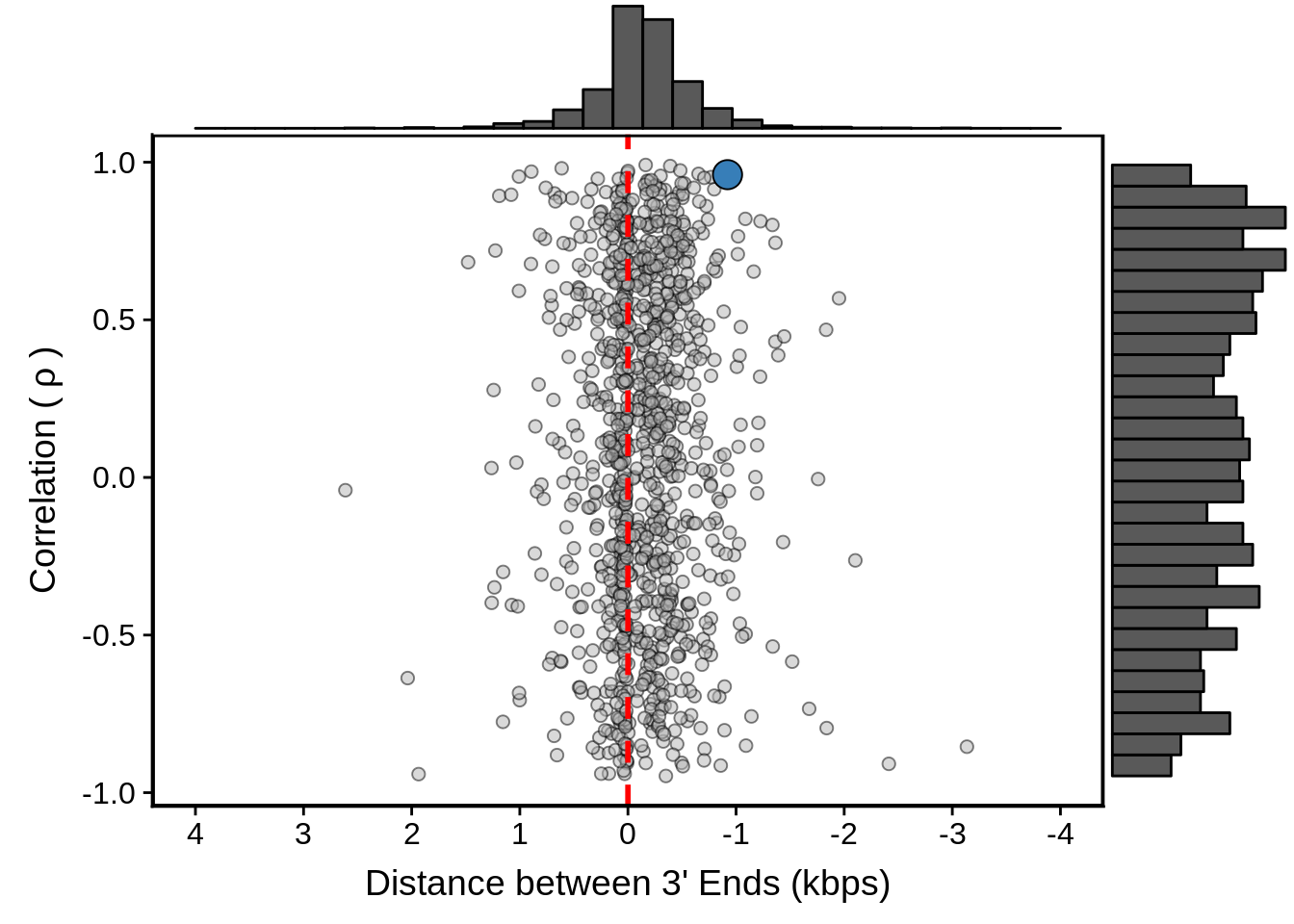Expand here to see past versions of 3d7tailtail-1.png: Version Author Date f0b9974 Philipp Ross 2018-09-23 f59e2e3 Philipp Ross 2018-09-22 ggsave(plot=g,filename="../output/neighboring_genes/3d7_tailtail.svg",height=3,width=4) Then we can make the HB3 plots: # write summary to a file sink("../output/neighboring_genes/hb3_divergent_summary.txt") summary(fxhb3_divergent)  left_gene right_gene dist cor Length:1005 Length:1005 Min. :-2344 Min. :-0.9510 Class :character Class :character 1st Qu.: 330 1st Qu.: 0.0330 Mode :character Mode :character Median : 713 Median : 0.6427 Mean : 1051 Mean : 0.4212 3rd Qu.: 1520 3rd Qu.: 0.8935 Max. : 7437 Max. : 0.9992 orientation Length:1005 Class :character Mode :character  sink(NULL) # plot results g <- fxhb3_divergent %>% ggplot(aes(x=dist,y=cor)) + geom_point(fill="grey70",color="black",pch=21,size=2,alpha=0.5) + panel_border(colour="black",size=1) + ylab(expression("Correlation ("~rho~")")) + xlab("Distance between 5' ends (kbps)") + scale_x_reverse(limits=c(8000,-3000), breaks=c(8000,7000,6000,5000,4000,3000,2000,1000,0,-1000,-2000,-3000), labels=c("8","7","6","5","4","3","2","1","0","-1","-2","-3")) + geom_vline(xintercept=0,linetype=2,col="red",size=1) + geom_point(data=subset(x3d7_divergent,left_gene=="PF3D7_1011900"&right_gene=="PF3D7_1012000"),fill="#E41A1C",color="black",pch=21,size=5) g <- ggExtra::ggMarginal(g, type = "histogram") print(g)Expand here to see past versions of hb3headhead-1.png: Version Author Date f0b9974 Philipp Ross 2018-09-23 f59e2e3 Philipp Ross 2018-09-22 ggsave(plot=g,filename="../output/neighboring_genes/hb3_headhead.svg",height=3,width=4) # write summary to a file sink("../output/neighboring_genes/hb3_convergent_summart.txt") summary(fxhb3_convergent)  left_gene right_gene dist Length:814 Length:814 Min. :-2491.00 Class :character Class :character 1st Qu.: -279.75 Mode :character Mode :character Median : 23.00 Mean : -31.44 3rd Qu.: 165.25 Max. : 7494.00 cor orientation Min. :-0.9564 Length:814 1st Qu.:-0.3743 Class :character Median : 0.2185 Mode :character Mean : 0.1552 3rd Qu.: 0.6882 Max. : 0.9963  sink(NULL) # plot results g <- fxhb3_convergent %>% ggplot(aes(x=dist,y=cor)) + geom_point(fill="grey70",color="black",pch=21,size=2,alpha=0.5) + panel_border(colour="black",size=1) + ylab(expression("Correlation ("~rho~")")) + xlab("Distance between 3' ends (kbps)") + scale_x_reverse(limits=c(8000,-3000), breaks=c(8000,7000,6000,5000,4000,3000,2000,1000,0,-1000,-2000,-3000), labels=c("8","7","6","5","4","3","2","1","0","-1","-2","-3")) + geom_vline(xintercept=0,linetype=2,col="red",size=1) + geom_point(data=subset(x3d7_convergent,left_gene=="PF3D7_1102700"&right_gene=="PF3D7_1102800"),fill="#E41A1C",color="black",pch=21,size=5) g <- ggExtra::ggMarginal(g, type = "histogram") print(g)Expand here to see past versions of hb3tailtail-1.png: Version Author Date f0b9974 Philipp Ross 2018-09-23 f59e2e3 Philipp Ross 2018-09-22 ggsave(plot=g,filename="../output/neighboring_genes/hb3_tailtail.svg",height=3,width=4) And finally for IT: # write summary to a file sink("../output/neighboring_genes/it_divergent_summary.txt") summary(fxit_divergent)  left_gene right_gene dist cor Length:942 Length:942 Min. :-4477.0 Min. :-0.9243 Class :character Class :character 1st Qu.: 355.2 1st Qu.: 0.1205 Mode :character Mode :character Median : 762.5 Median : 0.6316 Mean : 1141.9 Mean : 0.4459 3rd Qu.: 1699.8 3rd Qu.: 0.8848 Max. : 7426.0 Max. : 0.9999 orientation Length:942 Class :character Mode :character  sink(NULL) # plot results g <- fxit_divergent %>% ggplot(aes(x=dist,y=cor)) + geom_point(fill="grey70",color="black",pch=21,size=2,alpha=0.5) + panel_border(colour="black",size=1) + ylab(expression("Correlation ("~rho~")")) + xlab("Distance between 5' ends (kbps)") + scale_x_reverse(limits=c(8000,-5000), breaks=c(8000,7000,6000,5000,4000,3000,2000,1000,0,-1000,-2000,-3000,-4000,-5000), labels=c("8","7","6","5","4","3","2","1","0","-1","-2","-3","-4","-5")) + geom_vline(xintercept=0,linetype=2,col="red",size=1) + geom_point(data=subset(x3d7_divergent,left_gene=="PF3D7_1011900"&right_gene=="PF3D7_1012000"),fill="#4DAF4A",color="black",pch=21,size=5) g <- ggExtra::ggMarginal(g, type = "histogram") print(g)Expand here to see past versions of itheadhead-1.png: Version Author Date f0b9974 Philipp Ross 2018-09-23 f59e2e3 Philipp Ross 2018-09-22 ggsave(plot=g,filename="../output/neighboring_genes/it_headhead.svg",height=3,width=4) # write summary to a file sink("../output/neighboring_genes/it_convergent_summary.txt") summary(fxit_convergent)  left_gene right_gene dist cor Length:779 Length:779 Min. :-2118.0 Min. :-0.9810 Class :character Class :character 1st Qu.: -128.0 1st Qu.:-0.3282 Mode :character Mode :character Median : 70.0 Median : 0.1520 Mean : 104.9 Mean : 0.1503 3rd Qu.: 253.5 3rd Qu.: 0.6782 Max. : 4603.0 Max. : 0.9951 orientation Length:779 Class :character Mode :character  sink(NULL) # plot results g <- fxit_convergent %>% ggplot(aes(x=dist,y=cor)) + geom_point(fill="grey70",color="black",pch=21,size=2,alpha=0.5) + panel_border(colour="black",size=1) + ylab(expression("Correlation ("~rho~")")) + xlab("Distance between 3' ends (bps)") + scale_x_reverse(limits=c(5000,-3000), breaks=c(5000,4000,3000,2000,1000,0,-1000,-2000,-3000), labels=c("5","4","3","2","1","0","-1","-2","-3")) + geom_vline(xintercept=0,linetype=2,col="red",size=1) + geom_point(data=subset(x3d7_convergent,left_gene=="PF3D7_1102700"&right_gene=="PF3D7_1102800"),fill="#4DAF4A",color="black",pch=21,size=5) g <- ggExtra::ggMarginal(g, type = "histogram") print(g)Expand here to see past versions of ittailtail-1.png: Version Author Date f0b9974 Philipp Ross 2018-09-23 f59e2e3 Philipp Ross 2018-09-22 ggsave(plot=g,filename="../output/neighboring_genes/it_tailtail.svg",height=3,width=4) ### Individual profile plots We need to scale the data for appropriate plotting: fx3d7_abund <- x3d7_abund %>% dplyr::filter(gene_id %in% fx3d7) %>% dplyr::select(gene_id,tp,TPM) %>% dplyr::group_by(gene_id) %>% dplyr::summarise(m=mean(TPM)) %>% dplyr::inner_join(x3d7_abund) %>% dplyr::mutate(norm_tpm=(((TPM/m)-mean(TPM/m))/sd(TPM/m))) %>% dplyr::select(gene_id,tp,norm_tpm) %>% dplyr::ungroup() %>% tidyr::spread(tp,norm_tpm) sx3d7_abund <- fx3d7_abund %>% dplyr::rename(8=2, 16=3, 24=4, 32=5, 40=6, 48=7) %>% tidyr::gather(tp,norm_tpm,-gene_id) %>% dplyr::mutate(tp=as.numeric(tp)) require(org.Pf.plasmo.db) gene_names <- as.data.frame(org.Pf.plasmoGENENAME) plot_paired_profiles <- function(df, gid1, gid2) { df %>% dplyr::filter(gene_id == gid1 | gene_id == gid2) %>% ggplot(aes(x = tp, y = norm_tpm, color = gene_id,group=gene_id)) + ggtitle(paste(gid1,"\n ",gene_names[gene_names$gene_id==gid1,]$gene_name,"\n", gid2,"\n ",gene_names[gene_names$gene_id==gid2,]$gene_name)) + stat_smooth(se = F, size = 1.5) + geom_point(aes(fill=gene_id),color="black",pch=21,size=2) + scale_x_continuous(breaks = c(1,8,16,24,32,40,48), labels = c("T1", "T2", "T3" ,"T4", "T5", "T6", "T7")) + panel_border(colour="black",remove=F) + scale_color_brewer(palette="Paired") + scale_fill_brewer(palette="Paired") + ylab("Normalized TPM") + xlab("") + theme(legend.position="bottom") + geom_hline(yintercept=0,linetype=2,color="black",size=1) } #### Convergent example #plot_paired_profiles(sx3d7_abund,"PF3D7_1431300","PF3D7_1431400") #plot_paired_profiles(sx3d7_abund,"PF3D7_0214900","PF3D7_0215000") g <- plot_paired_profiles(sx3d7_abund,"PF3D7_1115900","PF3D7_1116000") ggsave(plot=g,filename="../output/neighboring_genes/convergent_pair.svg",width=4,height=4) print(g)Expand here to see past versions of unnamed-chunk-15-1.png: Version Author Date f59e2e3 Philipp Ross 2018-09-22 #### Divergent example g <- plot_paired_profiles(sx3d7_abund,"PF3D7_1011900","PF3D7_1012000") ggsave(plot=g,filename="../output/neighboring_genes/divergent_pair.svg",width=4,height=4) print(g)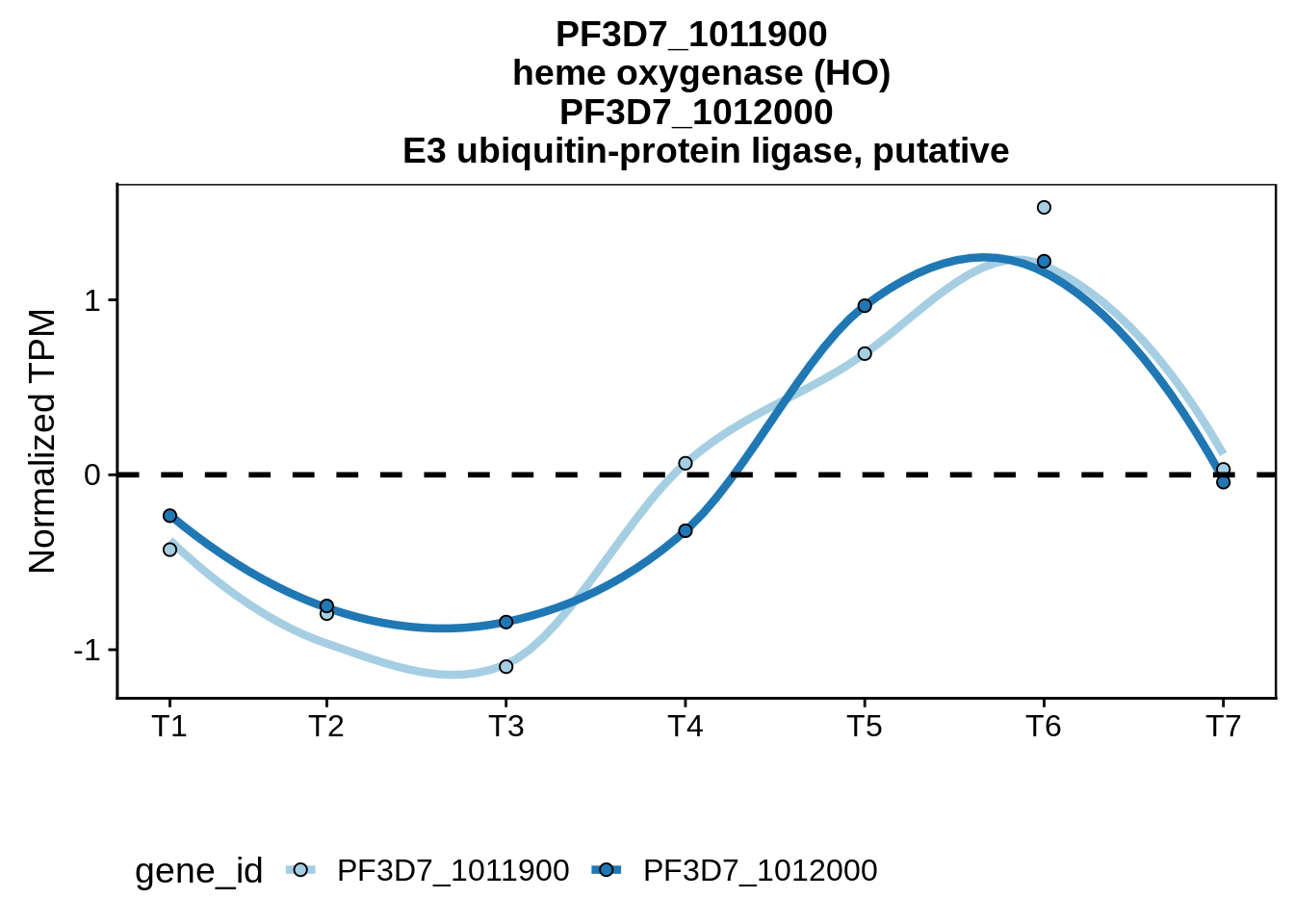Expand here to see past versions of unnamed-chunk-17-1.png: Version Author Date f0b9974 Philipp Ross 2018-09-23 f59e2e3 Philipp Ross 2018-09-22 ### Bidirectional promoters What if we split up the divergent neighboring genes by the distance separating them and plot their correlations individually. Do we see anything interesting? tmp <- fx3d7_divergent tmp$group <- dplyr::case_when(
tmp$dist <= 0 ~ "< 0", tmp$dist <= 500 & tmp$dist > 0 ~ "0-500", tmp$dist <= 1000 & tmp$dist > 500 ~ "500-1000", tmp$dist <= 1500 & tmp$dist > 1000 ~ "1000-1500", tmp$dist <= 2000 & tmp$dist > 1500 ~ "1500-2000", tmp$dist > 2000 ~ "> 2000"
)
tmp$group <- factor(tmp$group, levels=c("< 0","0-500","500-1000","1000-1500","1500-2000","> 2000"))
tmp %>% group_by(group) %>% summarise(m=mean(cor))
# A tibble: 6 x 2
group          m
<fct>      <dbl>
1 < 0       0.568
2 0-500     0.494
3 500-1000  0.337
4 1000-1500 0.0937
5 1500-2000 0.176
6 > 2000    0.123 
summary(tmp)
  left_gene          right_gene             dist            cor
Length:1119        Length:1119        Min.   :-2869   Min.   :-0.947766
Class :character   Class :character   1st Qu.:  229   1st Qu.:-0.005024
Mode  :character   Mode  :character   Median :  548   Median : 0.493932
Mean   :  857   Mean   : 0.351075
3rd Qu.: 1283   3rd Qu.: 0.784766
Max.   :10276   Max.   : 0.999899
orientation              group
Length:1119        < 0      :115
Class :character   0-500    :417
Mode  :character   500-1000 :225
1000-1500:137
1500-2000: 97
> 2000   :128  
g <- tmp %>% ggplot(aes(x=group,y=cor,group=group)) +
geom_boxplot(fill="#7FC97F",size=1) +
geom_hline(yintercept=mean(random_cor),linetype=2,col="red",size=1) +
theme(axis.text.x = element_text(angle=60, hjust=1)) +
xlab("") +
ylab(expression("Correlation ("~rho~")"))

ggsave(plot=g,filename="../output/neighboring_genes/divergent_groups.pdf",height=3,width=4)

print(g)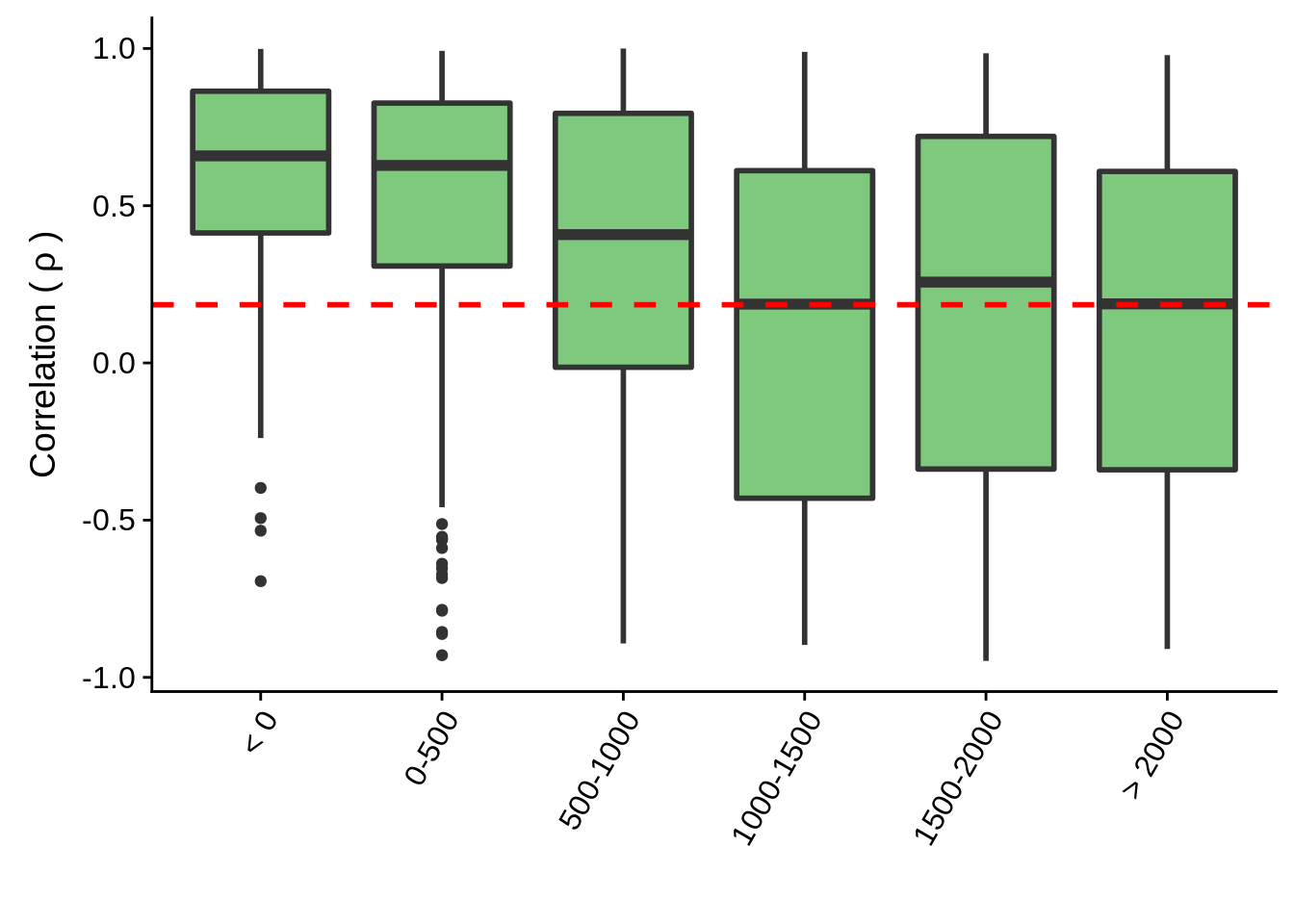Expand here to see past versions of unnamed-chunk-18-1.png:
Version Author Date
f0b9974 Philipp Ross 2018-09-23
f59e2e3 Philipp Ross 2018-09-22

Now to make a table of predicted bidirectional promoters:

bidirectional <- fx3d7_divergent %>%
dplyr::filter(dist<=1000,cor>=0.5) %>%
dplyr::select(-orientation) %>%
dplyr::inner_join(tibble::as_tibble(as.data.frame(org.Pf.plasmoGENENAME)),by=c("left_gene"="gene_id")) %>%
dplyr::rename(left_gene_name=gene_name) %>%
dplyr::inner_join(tibble::as_tibble(as.data.frame(org.Pf.plasmoGENENAME)),by=c("right_gene"="gene_id")) %>%
dplyr::rename(right_gene_name=gene_name) %>%
dplyr::select(left_gene,left_gene_name,right_gene,right_gene_name,dist,cor)

readr::write_tsv(x=bidirectional,path="../output/neighboring_genes/bidirectional_promoters.tsv")

### Transcriptional interference

tmp <- fx3d7_convergent
tmp$group <- dplyr::case_when( tmp$dist < -1000 ~ "< -1000",
tmp$dist >= -1000 & tmp$dist < -500 ~ "-1000--500",
tmp$dist >= -500 & tmp$dist < 0 ~ "-500-0",
tmp$dist >= 0 & tmp$dist < 500 ~ "0-500",
tmp$dist >= 500 & tmp$dist < 1000 ~ "500-1000",
tmp$dist > 1000 ~ "> 1000" ) tmp$group <- factor(tmp\$group, levels=c("< -1000","-1000--500","-500-0","0-500","500-1000","> 1000"))
tmp %>% group_by(group) %>% summarise(m=mean(cor))
# A tibble: 6 x 2
group           m
<fct>       <dbl>
1 < -1000    0.0394
2 -1000--500 0.0991
3 -500-0     0.126
4 0-500      0.0694
5 500-1000   0.160
6 > 1000     0.0189
summary(tmp)
  left_gene          right_gene             dist
Length:1059        Length:1059        Min.   :-3135.0
Class :character   Class :character   1st Qu.: -376.0
Mode  :character   Mode  :character   Median : -124.0
Mean   : -125.1
3rd Qu.:   69.5
Max.   : 7139.0
cor           orientation               group
Min.   :-0.94701   Length:1059        < -1000   : 37
1st Qu.:-0.35060   Class :character   -1000--500:128
Median : 0.11516   Mode  :character   -500-0    :433
Mean   : 0.09809                      0-500     :390
3rd Qu.: 0.58076                      500-1000  : 49
Max.   : 0.99123                      > 1000    : 22  
g <- tmp %>% ggplot(aes(x=group,y=cor,group=group)) +
geom_boxplot(fill="#BEAED4",size=1) +
geom_hline(yintercept=mean(random_cor),linetype=2,col="red",size=1) +
theme(axis.text.x = element_text(angle=60, hjust=1)) +
xlab("") +
ylab(expression("Correlation ("~rho~")"))

ggsave(plot=g,filename="../output/neighboring_genes/convergent_groups.pdf",height=3,width=4)

print(g)## Session information

sessionInfo()
R version 3.5.1 (2018-07-02)
Platform: x86_64-pc-linux-gnu (64-bit)
Running under: Gentoo/Linux

Matrix products: default
BLAS: /usr/local/lib64/R/lib/libRblas.so
LAPACK: /usr/local/lib64/R/lib/libRlapack.so

locale:
 LC_CTYPE=en_US.UTF-8       LC_NUMERIC=C
 LC_TIME=en_US.UTF-8        LC_COLLATE=en_US.UTF-8
 LC_MONETARY=en_US.UTF-8    LC_MESSAGES=en_US.UTF-8
 LC_PAPER=en_US.UTF-8       LC_NAME=C
 LC_MEASUREMENT=en_US.UTF-8 LC_IDENTIFICATION=C

attached base packages:
 parallel  stats4    stats     graphics  grDevices utils     datasets
 methods   base

other attached packages:
 gdtools_0.1.7
 bindrcpp_0.2.2
 BSgenome.Pfalciparum.PlasmoDB.v24_1.0
 BSgenome_1.48.0
 rtracklayer_1.40.6
 Biostrings_2.48.0
 XVector_0.20.0
 GenomicRanges_1.32.7
 GenomeInfoDb_1.16.0
 org.Pf.plasmo.db_3.6.0
 AnnotationDbi_1.42.1
 IRanges_2.14.12
 S4Vectors_0.18.3
 Biobase_2.40.0
 BiocGenerics_0.26.0
 scales_1.0.0
 cowplot_0.9.3
 magrittr_1.5
 forcats_0.3.0
 stringr_1.3.1
 dplyr_0.7.6
 purrr_0.2.5
 tidyr_0.8.1
 tibble_1.4.2
 ggplot2_3.0.0
 tidyverse_1.2.1

loaded via a namespace (and not attached):
 nlme_3.1-137                bitops_1.0-6
 matrixStats_0.54.0          lubridate_1.7.4
 bit64_0.9-7                 RColorBrewer_1.1-2
 httr_1.3.1                  rprojroot_1.3-2
 tools_3.5.1                 backports_1.1.2
 utf8_1.1.4                  R6_2.3.0
 DBI_1.0.0                   lazyeval_0.2.1
 colorspace_1.3-2            withr_2.1.2
 tidyselect_0.2.4            bit_1.1-14
 compiler_3.5.1              git2r_0.23.0
 cli_1.0.1                   rvest_0.3.2
 xml2_1.2.0                  DelayedArray_0.6.6
 labeling_0.3                digest_0.6.17
 Rsamtools_1.32.3            svglite_1.2.1
 rmarkdown_1.10              R.utils_2.7.0
 pkgconfig_2.0.2             htmltools_0.3.6
 rstudioapi_0.8              RSQLite_2.1.1
 shiny_1.1.0                 bindr_0.1.1
 jsonlite_1.5                BiocParallel_1.14.2
 R.oo_1.22.0                 RCurl_1.95-4.11
 GenomeInfoDbData_1.1.0      Matrix_1.2-15
 fansi_0.3.0                 Rcpp_0.12.19
 munsell_0.5.0               R.methodsS3_1.7.1
 stringi_1.2.4               whisker_0.3-2
 yaml_2.2.0                  SummarizedExperiment_1.10.1
 zlibbioc_1.26.0             plyr_1.8.4
 grid_3.5.1                  blob_1.1.1
 promises_1.0.1              crayon_1.3.4
 miniUI_0.1.1.1              lattice_0.20-38
 haven_1.1.2                 hms_0.4.2
 knitr_1.20                  pillar_1.3.0
 XML_3.98-1.16               glue_1.3.0
 evaluate_0.11               modelr_0.1.2
 httpuv_1.4.5                cellranger_1.1.0
 gtable_0.2.0                assertthat_0.2.0
 ggExtra_0.8                 mime_0.5
 xtable_1.8-3                broom_0.5.0
 later_0.7.5                 GenomicAlignments_1.16.0
 memoise_1.1.0               workflowr_1.1.1            

This reproducible R Markdown analysis was created with workflowr 1.1.1